Categories

# What you should know for SAT Math

The November and December SATs are around the corner! Here is a list of topics that are frequently tested on the SAT.

## Finding x and y intercepts

To find the x-intercept, plug zero into y and solve. To find the y-intercept, plug zero into x and solve. You do NOT need to isolate the variable first. Do not do this. This often takes more time.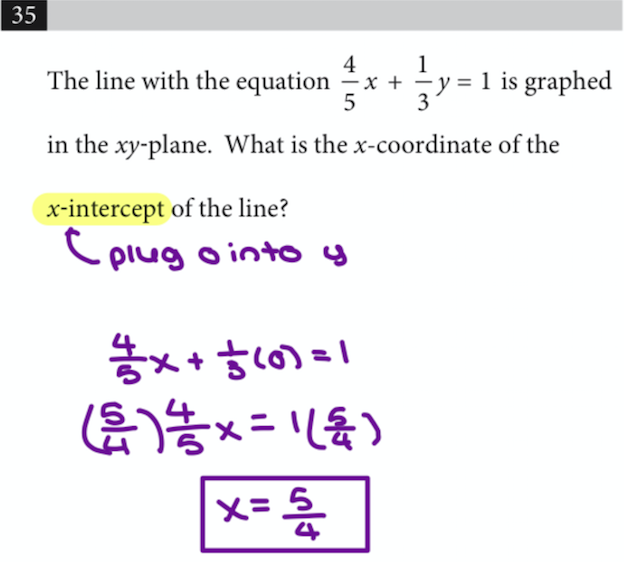For exponential functions, REMEMBER ANYTHING TO THE ZEROTH POWER IS ONE! This will help when you need to find the y-intercept.## Circle Equations

You should know how to find the center and radius of a circle in expanded form. If you need to learn how to do this, check out Khan Academy!## Sum of the roots

If the SAT wants to know the sum of the solutions of a quadratic, you do not need to find the individual solutions!! You can use the formula sum = -b/a. Often you’ll have to use the quadratic formula to find the roots, which takes an enormous amount of time.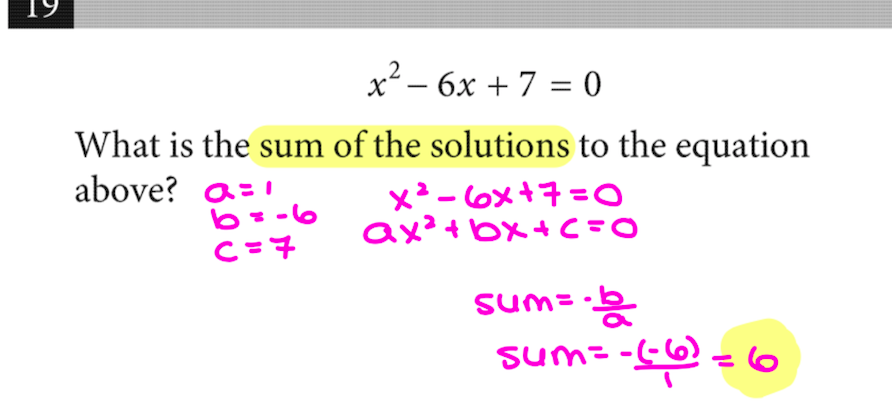## Linear systems with no / infinite solutions

Linear systems or equations with no or infinite solutions mean the x terms should have the same coefficients! If they have the same coefficients, the x values will cancel. Additionally, you can think of them as having the same slope. The same slope means parallel lines.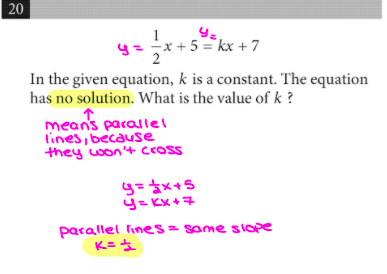## Solving Systems by elimination

Yes, I know that you can use substitution and other methods. However, I’ve found that elimination is the most efficient. Substitution can lead to careless errors from distribution or fractions. Also, the equations are often set up so that elimination is faster. Here is some elimination practice!## Standard Deviation

Standard deviation measures the spread. In general, the bigger the range the bigger the standard deviation. If the ranges are equal, look for the middle of the data. The more numbers clustered around the middle, the smaller the standard deviation. I hope that helps!## Box Plots

The most important thing to know about box plots is that the middle line is the median. You CANNOT determine the mean (or average) from a box plot.It seems like almost every test has one non-calculator question that requires the quadratic formula (or complete the square, but the quadratic formula is easier for most students). Therefore, I strongly recommend memorizing and being familiar with using the formula. Here is an example question that showed up in a recent non-calculator section.Here is some extra practice!

## Linear systems shortcuts

If a linear system of equations is not asking you to find the x and y solutions individually, look for a shortcut! Sometimes, you don’t have to find (x,y) to get the answer. For example, if the question wants 5x + 5y, see if you can add or subtract the two equations to get it to appear. You can save an enormous amount of time doing this! Here are two recent examples: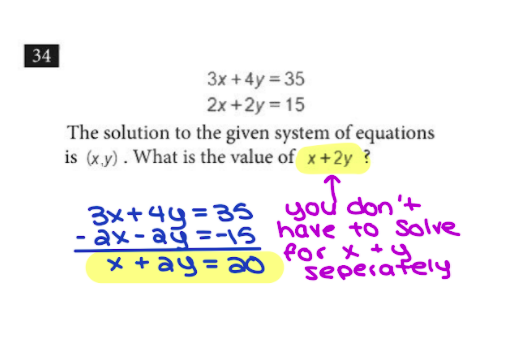## Finding the equation of graphs

If you need to find the equation of a graph or scatterplot, plug in a point from the graph into the equations. Whichever answer’s equation works best with the point is the answer. I recommend picking one of the later points. If it’s a scatterplot, I recommend sketching in a best-fit curve or line and picking a ‘nice’ (whole number coordinates) point from that line/curve. Again, whichever answer’s equation works with the point will be the answer. It’s important to plug the point into all four answers to make sure one only works! If more than one equation works, use another point.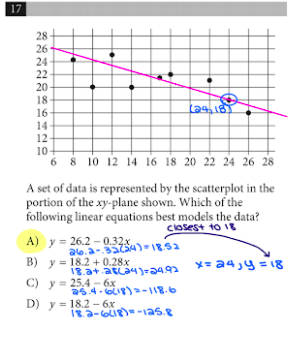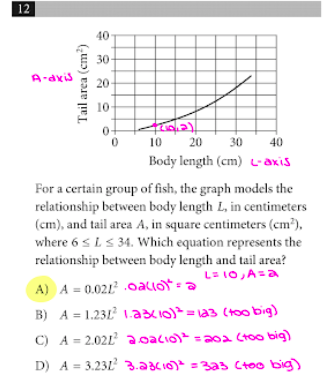There are more topics, but these are the first that came to mind. A couple more facts to remember:

• Parallel lines = same slope, perpendicular lines = negative reciprocal (flipped fraction) slope.
• i2 = -1
• Geometry figures will be drawn to scale unless otherwise specified. If you can’t solve it, estimate the answer based on the picture!
• When sin x = cos y, then angles x and y add to 90 degrees.
• Make sure your calculator is charged and use it! Here is a video of super helpful SAT calculator tricks.

If you think I left any other frequently tested topics, please comment or send us a message!